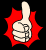# Moments SummaryThe turning effect (or moment) of a force is given by:
moment = force × perpendicular distance from pivotThe normal units used for force and distance are newtons and metres respectively, so the usual unit for moment is the newton-metre (Nm)Another name for a pivot is fulcrum.Moments can either be clockwise or anticlockwise.When more than one force acts in the same direction, their overall turning effect is just the sum of their moments.When forces act in a different direction, yet still balance, the total turning effect in each direction will be the same:
sum of clockwise moments = sum of anticlockwise momentsForces & Motion Menu